Part 1 – Promotion

Nowadays, human resources (HR) analytics are at the center of attention in academic research. With increased HR-related data availability, the use of statistical methods has gained greater importance. HR management is evolving to a more efficient system by analyzing and estimating related information.

In this paper, open-source HR data is used to estimate the probability of the promotion of a worker to a higher position using both statistics-econometrics and machine learning methods. The approach used here is closer to machine learning as the study focuses more on accurately predicting than interpreting the features. The statistical learning part is linked to the use of conventional and penalized logistic regressions. The borderline method is applied with the LASSO operator for both prediction and feature selection as inputs of machine learning methods.

The methods used are perfectly replicable for a given data set of any Azerbaijani entity. A similar method could be applied to analyze nepotism. Statistical machine learning can be used to assess the probability of a worker getting promoted depending on their personal connections. If a worker has a high probability of being promoted (or is estimated as will be promoted) but is not and instead someone with a lower probability is, it would be a sign of nepotism. Regional and gender dummies might be useful for this purpose as well. Section 2 will be dealing with different data sets to estimate the probability of leaving a job for a given worker.

Data and Methodology

The data collected for this study includes 50,000 observations with around 20 initial features. The response variable shows whether a worker was promoted or not. More features were created by taking squares or cubes of the suitable initial feature but there was no gain in predictive power.

Moreover, the standardization and normalization of variables was tried but no gain was obtained.  As a result, the variables are used in simple level forms except for the KNN estimator. Around 8% of the data has a promotion tag equal to 1. The data was split and trained in a 30/70 ratio between test and training sets for model accuracy.  This split ratio was chosen to avoid significant losses.

Starting with a simple conventional logistic regression and 11 penalized shrinkage logistic regressions, 11 models were returned by tuning the alpha parameter from 0 to 1 with intervals of 0.1. When alpha equals zero, the regression is called Ridge. When it equals 1, it is called LASSO. Any value in between would be called elastic-net, especially when alpha is equal to 0.5. The alpha parameter is further discussed later in this paper.

These models are executed on the training set and get their AUROC  (simply AUC from now on) for comparison. Statistically significant features are chosen based on their p-value for simple conventional logistic regression. All features are input for shrinkage models to decide which features to keep and which ones to shrink to zero due to insignificant values. It should be noted that the Ridge regression where the alpha parameter is set to zero keeps all features.

Results for Statistical Learning

1) Simple conventional logistic regression results.

The AUC for this model over the training set is 0.8735. This value is used as a benchmark for the following steps. 15 features are returned and the pseudo-R2 is around 0.32, which is a sign of good fit for a logistic regression. Shrinkage logistic regressions are applied using the glmnet package of R programming code. The chosen alpha parameter is between 0 and 1 with an interval of 0.1 that returns 11 models.

Table 1. Results for Penalized Logistic Regressions

 alpha AUC 0 0.8654773 0.1 0.8765511 0.2 0.8770106 0.3 0.8766488 0.4 0.8767241 0.5 0.8765327 0.6 0.8770255 0.7 0.8764979 0.8 0.8767012 0.9 0.8764419 1 0.8765889

Table 1 reveals the three best models according to their AUC and respective alpha parameter. All three models can be labelled as elastic-net regressions and used to choose the model with the highest predictive power on the unseen test data. Although the numbers are in close range to each other. Graph 1 shows how volatile the prediction power is.

It should be noted that only a Ridge regression with alpha value 0 underperforms benchmark conventional logistic regression, justifying the use of modern shrinkage logistic regressions in this study. Moreover, shrinkage models overcome ill-conditioned cases such as highly correlated variables or separation problems resulting in lower prediction error.

Graph 1. AUCs of Training Set ModelsThe study’s test set includes the following values: 0 and 1. However, the models predict probabilities between zero and one. A function predicting classes could be used but would make the program choose the cutoff value for probabilities as 0.5, which is not justified due to oversimplification. A cutoff value of 0.5 means above 0.5 class is predicted as 1, otherwise as 0.

Criteria and model comparison must be introduced to justify such a cutoff value. AUC can be used for the test set, but more universal criteria are required to make these models comparable with the machine learning counterparts.

Three concepts need to be defined: accuracy, sensitivity, and specificity. Accuracy is the portion that has been captured by the model correctly. If the accuracy is 90%, then 90% of the zeros and ones were predicted correctly. Sensitivity measures the rate at which a model correctly predicts true positive (TP) rates. On the contrary, specificity measures the rate at which a model correctly predicts negatives (0).

Three criteria are used to choose the cutoff values for each of the three models. The first is the cutoff that maximizes accuracy. The second maximizes both sensitivity and specificity, minimizing the distance between the upper left corner of ROC curve graph and the curve itself.

The last criterion is the cost-minimizing cutoff, which minimizes the self-defined cost function. This cost function sums up false negatives and false positives to achieve a situation where false negatives are twice as costly as false positives. In this case, the cost of labeling a promoted worker as not promoted is costlier than labeling a non-promoted worker as promoted.

Table 1: Results of selected 3 models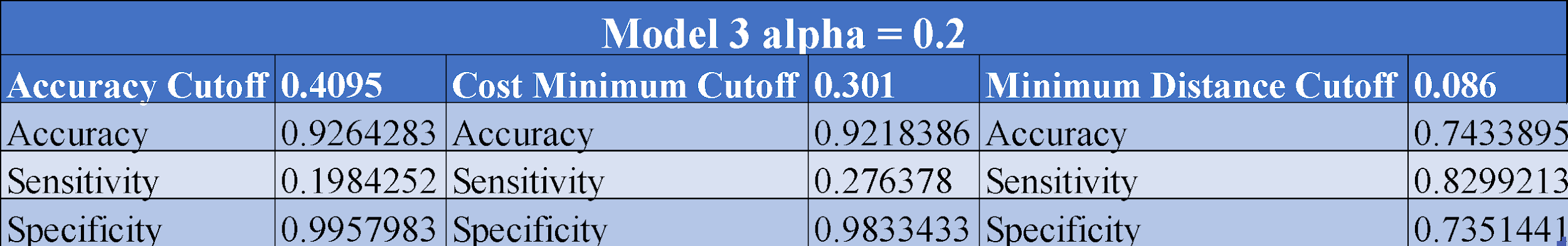Several observations need to be mentioned. First, the accuracy cutoffs achieve the highest accuracy as expected. If a cost minimizer cutoff were used instead, with very little loss on accuracy and specificity, sensitivity would have gained 40%.

However, by losing about 20% in accuracy and specificity, sensitivity is quadrupled. The focus of this study is to find which workers will be promoted (and not lose those workers). The model that achieves this best is model 7, with the highest sensitivity.

It should be noted that this result may vary depending on the situation or management decision. For example, in estimating loan loss provisions, if the minimal distance cutoff had been chosen, the prediction power in estimating loan loss provisions for borrowers failing to make their loan payments would have been achieved but at the cost of allocating more provisions.

For this study, a lower cutoff was preferred to gain higher predictive power for those who will be promoted at the cost of promoting more people. These models give the freedom to tune from one to another depending on our need and approach. Note that our cutoff value differs from the naive 0.5 points.

The following paragraphs will further analyze Model 7.

Graph 2. AUCs of the Model per Lambda Parameter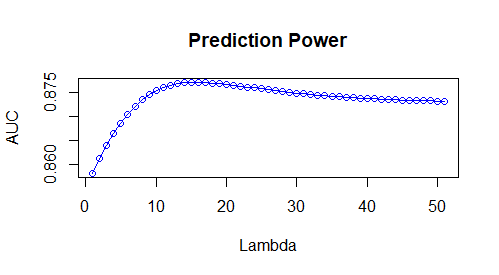Graph 3. ROC Curve of the Model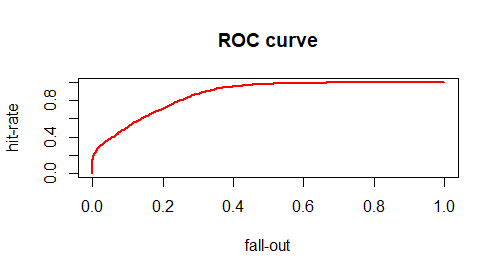In the graph above, the hit-rate is sensitivity and fall-out is false positive rate, which is 1-specificity. Thus, the minimal distance from upper left corner to the curve maximizes sensitivity and specificity as the uppermost left corner is the ideal point.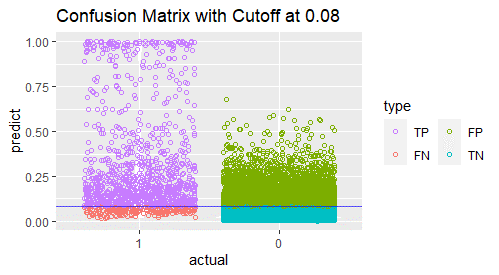The graph above shows a confusion matrix of suitable points for each category (True Positive, True Negative, False Positive, False Negative). The number of TPs is quite high since we chose the cutoff with highest sensitivity (hit-rate).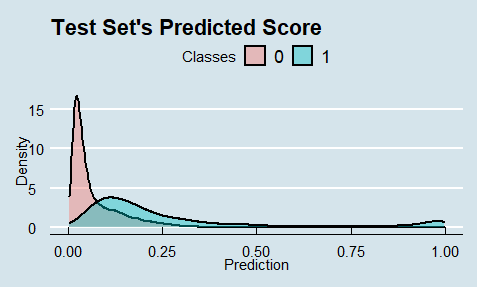The graph above shows the densities of predictions per class. A significant overlap is observed for the data given. Moreover, the graphs indicate that the minimal distance cutoff value 0.08 is reasonable.

Machine Learning Results

Several basic machine learning tools are used for further classifications. Unlike the previous ones, these methods yield classes (0 and 1) as predicted outcomes. Thus, there is no cutoff problem or otherwise decision freedom.

Other methods could have been used. For example, XGBOOST, Adaptive Lasso, and Firth regularization were possible options but did not result in any tangible improvement over the method used for this study. Some of these techniques are more time-consuming and require more computing power. Thus, the LASSO operator (where alpha is equal to 1) is used like in the models run before for feature selection.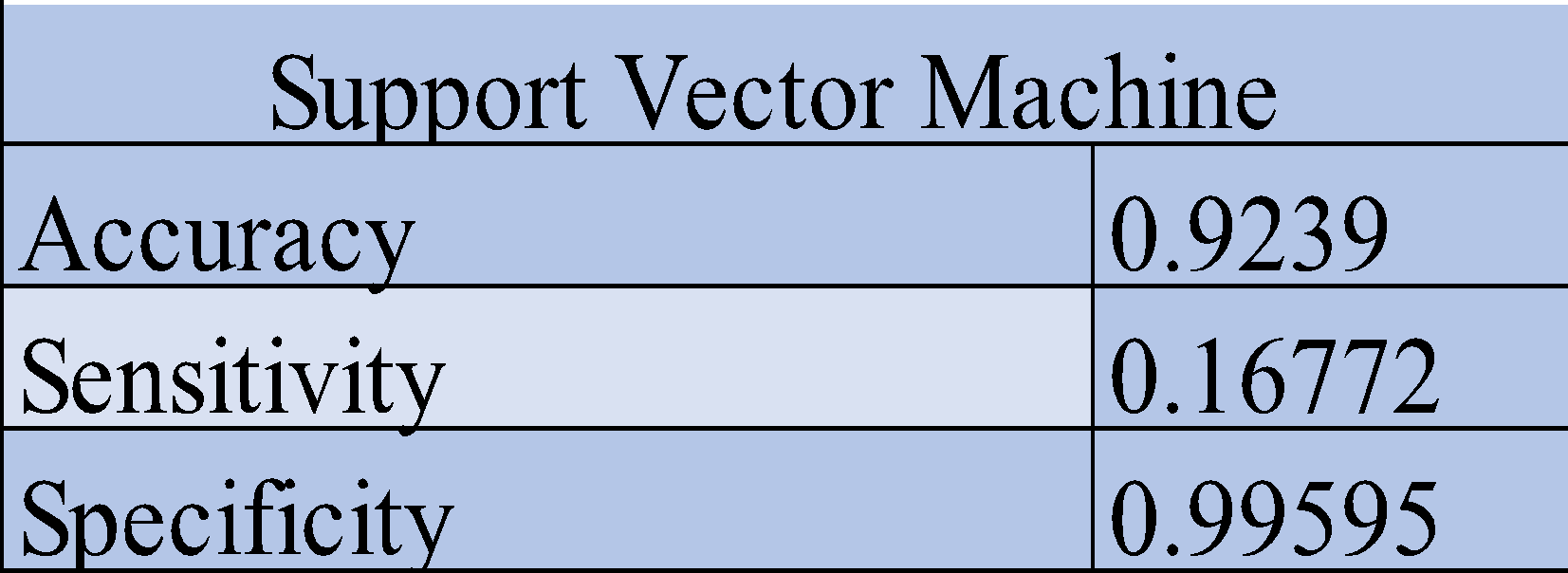The Support Vector Machine (SVM) results above are similar (albeit lower) to the best elastic-net model with accuracy maximizer cutoff.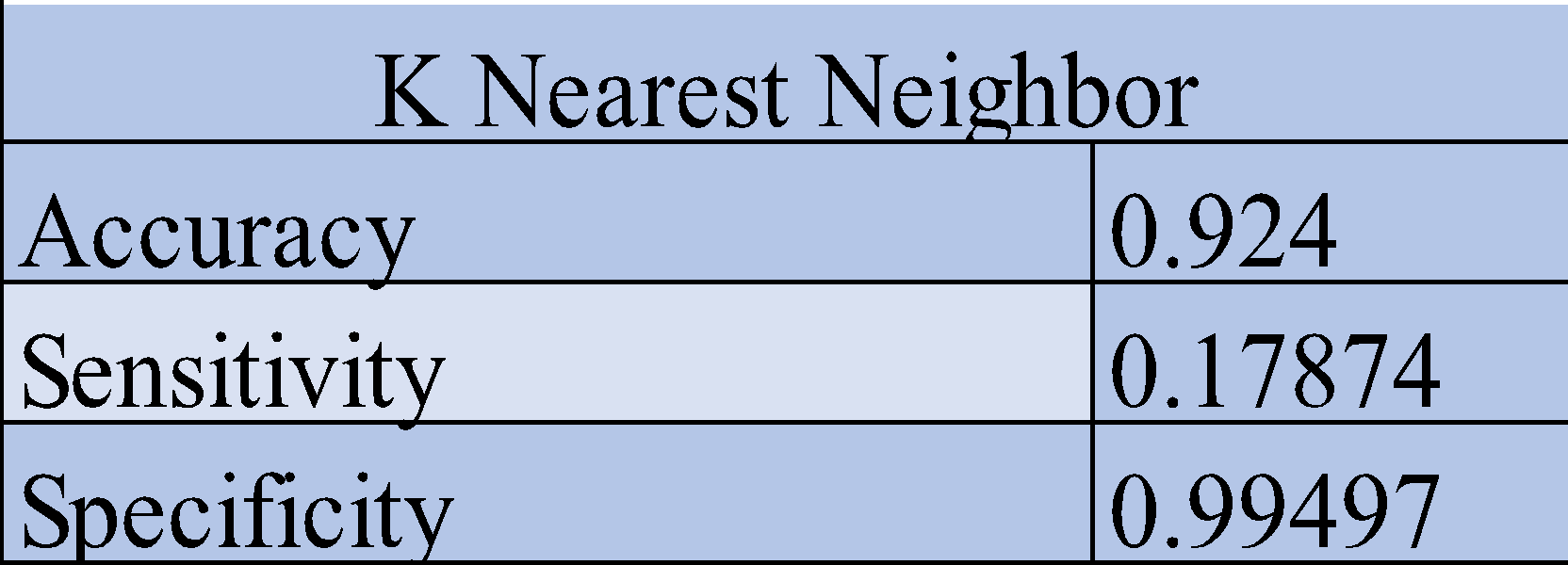The K Nearest Neighbor (KNN) model returns better results than SVM but yields much lower sensitivity than model 7.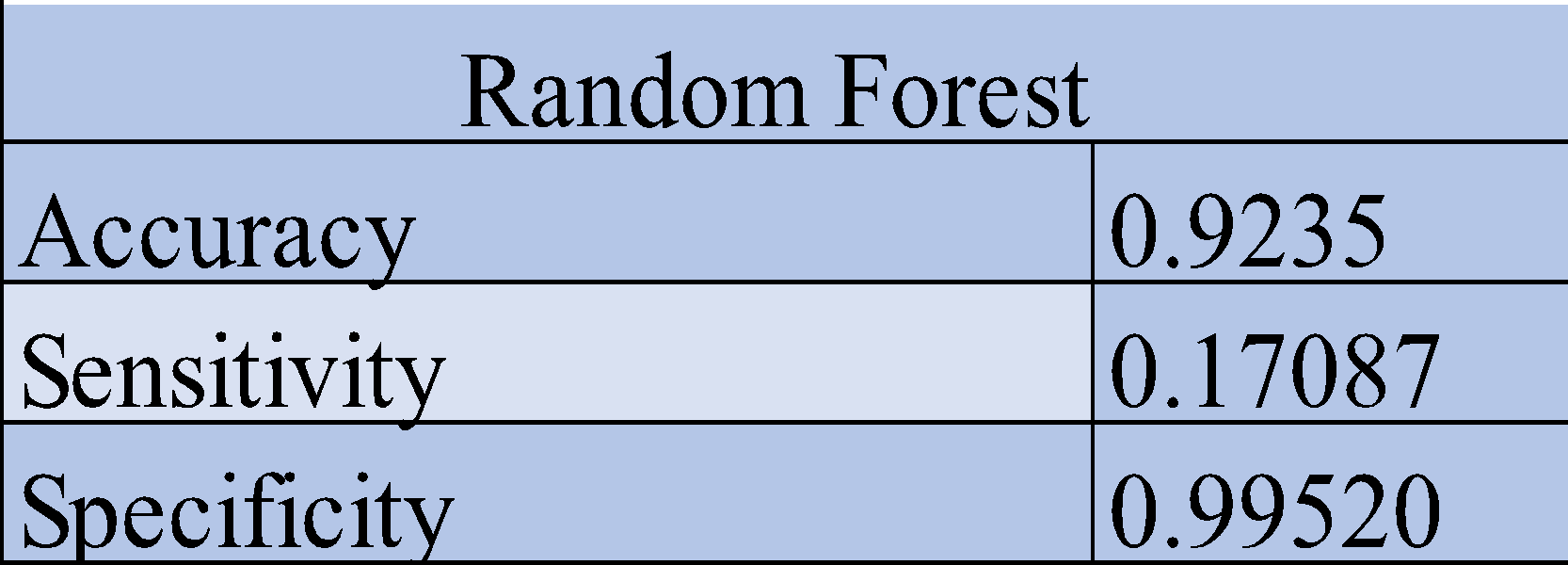The above also yields similar results with higher sensitivity than SVM but lower than KNN.The Naïve Bayes results are closer to those of model 7 and achieve minimum distance cutoff. Sensitivity here is much higher than in other pure machine learning counterparts.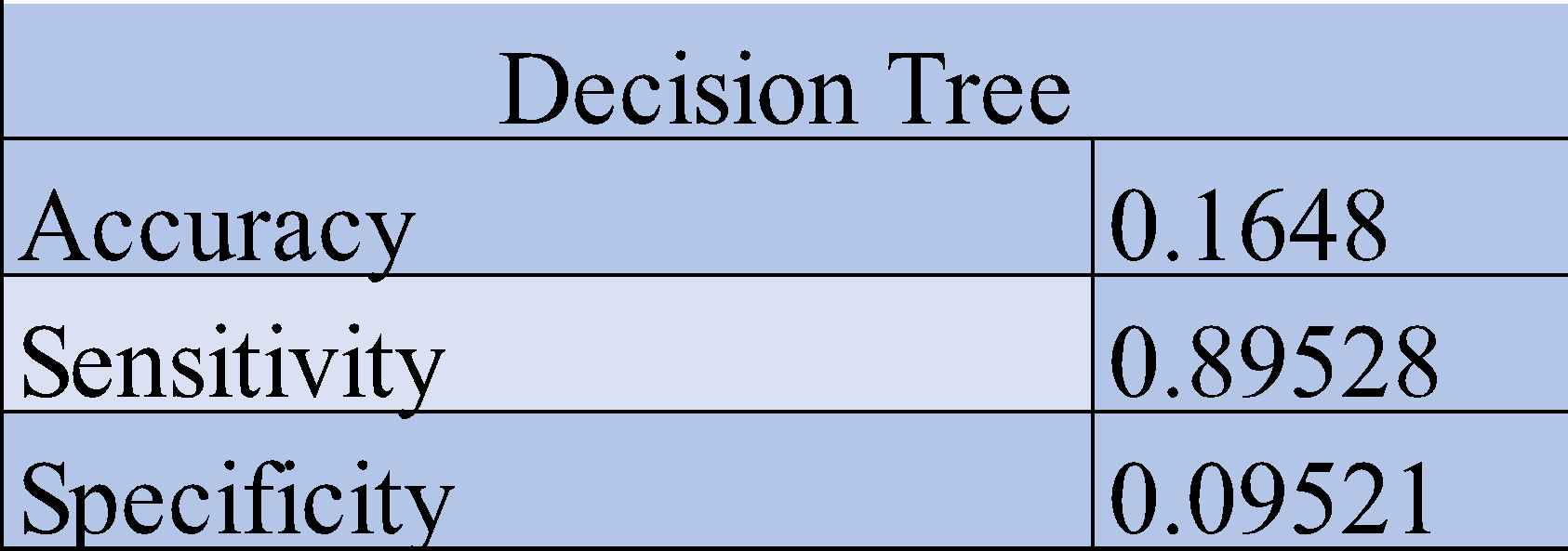This method yields the highest sensitivity out of all the models used in this study. Indeed, the sensitivity is better than Model 7 and achieves minimum distance cutoff. A more surprising observation shows that the accuracy and specificity are both unacceptably low. This model can capture class 1 with very high precision but cannot correctly capture class 0, highlighting the importance of using several criteria before choosing the best model.

Conclusion and Further Discussion

This study’s results show that the best model to estimate the probability of the promotion of a worker to a higher position is a regularized logistic model or a shrinkage model. The method used here is using both pure statistical learning and pure machine learning techniques. The results highlight the importance of comparing several models with different criteria to evaluate and justify each model reliably.

Further research can increase the choice for the alpha parameter and try, for example, 100 values (or more depending on the computing power and without losing the sense) and end up with many more models. One interesting issue to look at would be when (for which value of alpha) a feature starts to be picked up (or vice versa).

 KNN- k nearest neighbor

 AUROC – The area under the receiver operating characteristic